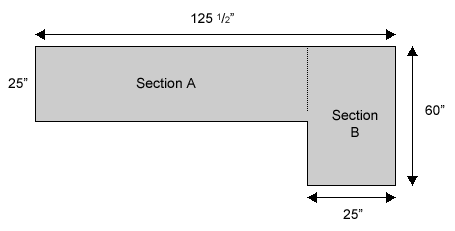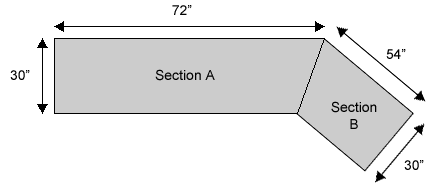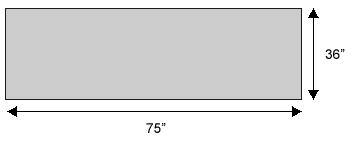# How to Measure Countertops

### L Shape Counter Top

#### To Measure Section A:

Take the wall dimension of Section A & minus the depth of Section B
125" – 25 " = 100"

Take this number & multiply by the depth of Section A
100" x 25" = 2500 sq. in.

Divide this number by 144
2500 ¸ 144 = 17.36 sq. ft.

#### To Measure Section B:

Take the wall dimension of Section B & multiply
by the depth of Section B
60" x 25 " = 1500 sq. in.

Divide this number by 144
1500 ¸ 144 = 10.41 sq ft.

Add the total of Section A & Section B & round to the nearest ¼ sq. ft.
17.36 + 10.41 = 27.77 sq. ft. / The total counter top is 28 sq. ft.### Angled Island Top

#### To Measure Section A:

Take the length of Section A & multiply by the depth of Section A
72" x 30" = 2160 sq. in.

Divide this number by 144
2160 ¸ 144 = 15.0 sq. ft.

#### To Measure Section B:

Take the length of Section B & multiply by the depth of Section B
54" x 30" = 1620 sq. in.

Divide this number by 144
1620 ¸ 144 = 11.25 sq. ft.

Add the total of Section A & Section B & round to the nearest ¼ sq. ft.
15.0 + 11.25 = 26.25 sq. ft. / The total counter top is 26 ¼" sq. ft.### Rectangular Island Top

Take the length of the top & multiply by the depth
75" x 36" = 2700 sq. in.

Take this number & divide by 144
2700 ¸ 144 = 18.75 sq. ft.

• Allow for overhang on any exposed end.
• If you are making dimension changes from existing countertops to new countertops, be sure to consider space needed to accommodate any extra length or width added.
• When ordering a U shaped countertop to fit between walls please be sure to allow room to slide the countertop in place, typically 1/4".### Standard Countertop Specifications:

#### Kitchen Countertops

Our 25-5/16" deep countertops fit on standard 24" deep kitchen cabinets.
An optional loose backsplash is available and comes at a standard 3" height.

#### Kitchen Island / Peninsulas

27" and 36" deep islands can be used on standard 24" deep kitchen cabinets as a double-sided workspace or a snack bar.

#### Bar Top

15" and 18" deep island / peninsula tops fit on half walls or elevated wall cabinets.

#### Vanity Countertops

19" and 22" deep countertops fit on 18" and 21" deep standard vanities.